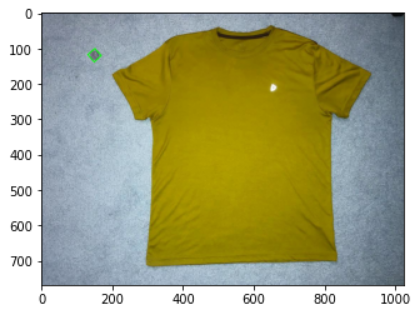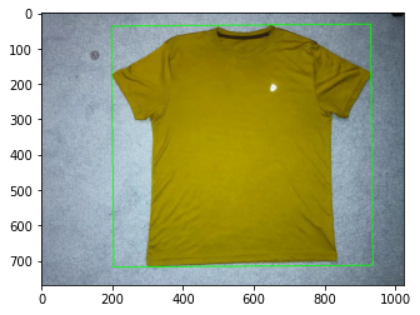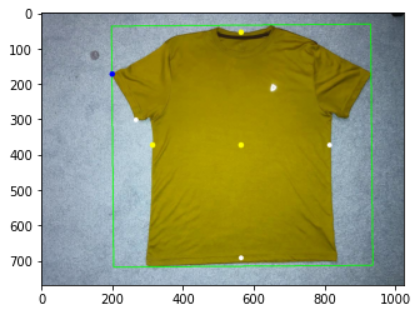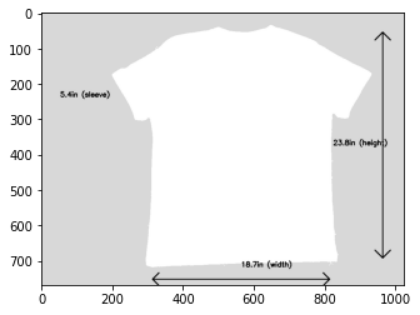# Fashion E-Commerce: Using Computer Vision to Find Clothing that Fits Like a Glove¶

Never let online trends get in the way of creating a great outfit for yourself.## Objective¶

In this tutorial, you'll learn to build an algorithm in Python that computes the measurements of a T-Shirt from an image. So roll up your sleeves, and follow along!

### Let's begin by first importing a few Python libaries¶

Here's some brief descriptions about the packages you'll rely on to build this program:

• cv2: Pre-built CPU-only OpenCV packages for Python. OpenCV (Open Source Computer Vision Library) is a library of programming functions mainly aimed at real-time computer vision.
• numpy: A scientific computing package for Python that adds support for large, multi-dimensional arrays and matrices, along with a large collection of high-level mathematical functions to operate on these arrays.
• matplotlib: A library for creating static, animated, and interactive visualizations in Python
• imutils: A series of convenience functions to make basic image processing functions such as translation, rotation, resizing, skeletonization, displaying Matplotlib images, sorting contours, detecting edges, and much more easier with OpenCV.
• scipy: Python-based ecosystem of open-source software for mathematics, science, and engineering.

Did you know? Numpy along with libraries like SciPy and Matplotlib enabled the Event Horizon telescope to produce the first ever image of a black hole

Good. Now that you have imported the libaries, let's begin by first importing an image of a T-Shirt. Assume that this T-Shirt is your current best-fit.

## Load the image of the T-shirt that is your current best fit¶

Use cv2.imread to read an image from your file system.

For knowing what the image looks like, you can use Matplotlib's imshow function, which will read the image, and then use show to display it.

You probably noticed that there is a circular object on the top left of this image. It's a US quarter, and it's there for a reason - to help determine the size of the outfit!

How? Let's understand the problem further.

# How do you measure the size of an object in an image?¶

Depending on how you take the photograph of the T-Shirt, it might appear smaller in the picture, or larger. So how would you know the true dimensions of the T-Shirt? Seems a bit complex right?

This ia a very common problem in applications that involve object and size detection. The most straight-forward and basic solution is to o define a ratio that measures the number of pixels per a given metric. For this, you'll use a reference object, an object that is uniquely identifiable in some manner. This object should have a couple of important properties:

1. Using computer vision, you should be able to easily find this object in the image. Here are some ways to do that:
• Place this object in the image always in the same place (for example, on the top-left corner). This is perhaps the easiest solution
• Use an object that's of a distinctive unique color, or a unique shape.
2. You should know the dimensions of this object in some measurable unit (for example, in centimetres, millimetres, or inches).

A US Quarter serves as a good reference object, because you already know it's size, and it's a shape that's unique so therefore easy to identify. You'll place it on the top left before clicking the photograph. Then, here's what you'll do:

1. You'll find contours for the image, and sort them from left-to-right.
2. Once sorted, the contour for the quarter will always be the first contour in the sorted list. You'll grab it and use it to calculate the number of pixels per a given metric. Here's the formula:

pixels_per_metric = object_width / known_width

A US quarter has a known_width of 0.955 inches. So if your object_width (measured in pixels) is computed be 150 pixels wide (based on its associated bounding box), the pixels_per_metric will be:

pixels_per_metric = 150px / 0.955in = 157px.

This means, that there are approximately 157 pixels per every 0.955 inches in our image. Using this ratio, we can compute the size of objects in an image.

Source: I found this excellent tutorial from PyImageSearch

Now that you've got the gist of it, let's begin coding!

# Image processing¶

### Convert the best-fit image to grayscale and apply some blur¶

Tip: At any point, if you need to know what a variable contains, or need to see what the results of a certain image processing technique, feel free to use Python's print function and Matplotlib's imshow and show methods

# What are Contours?¶

To put it simply, it's a curve joining all the continuous points (along the boundary), having same color or intensity. Contours are a useful tool for shape analysis and object detection and recognition

Now you have the contours, you'll sort them from left-to-right. Then you'll find the max of these contours, which is the area that your T-shirt occupies

## Find image moments¶

An image moment is a certain particular weighted average (moment) of the image pixels' intensities. It allows you to find image properties such as its area (or total intensity), its centroid, and information about its orientation.

## Examine the contours and calculate the outfit measurements¶

You'll loop over the contours in-order to examine them. On each loop:

1. First check if the contour area is sufficiently large. If it isn't then you'll ignore it, as it might be just noise from the edge-detection process.
2. Next, compute the rotated bounding box of the contour.
3. Then organize the points in the contour, such that they appear in top-left, top-right, bottom-right, and bottom-left order, and draw the outline of the bounding box.

Since the reference object is on the top-left, the first iteration will create an outline on the US quarter, and the 2nd iteration would create an outline on the T-Shirt, like this:1. Next, check if the pixels_per_metric variable is initialized. If it isn't, then you'll use the bounding box to calculate the euclidian distance in pixels. This will be the value of object_width in the pixels_per_metric formula

2. Next, you'll find the top and bottom points of the T-shirt, as well as the Top and Bottom points of the T-shirt sleeve. You'll need these in-order to calculate the width and the height of the T-shirt and also the width of the T-shirt sleeve:3. Finally, you'll label the measurements on the T-shirt!